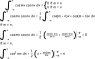# Trigonometric Orthogonality Query

• Emphacy

#### Emphacy

Hello everyone, I've wandered PF a few times in the past but never thought I'd join, here I am, how exciting.

To keep it short I'm trying to understand the proof behind Fourier Series and can't quite get to grips with basic trigonometric orthogonality.
I understand that sin and cos are naturally orthogonal but I'm having difficulty understanding this:

$$\int_{-\pi}^{\pi}\cos(mx)\cos(nx)\, dx=\pi\delta_{mn} \,\,\,where\,\delta_{mn}=\begin{cases} &\text{1 if } m=n \\ &\text{0 if } m\neq n \end{cases}$$

It makes sense that the area is $\pi$ if $m=n$ because you get this waveform and zero if m is an integer multiple of n because you get this waveform (here the negative areas cancel the positive areas from $-\pi$ to $\pi$)

BUT... If $m\neq n$ AND is sufficiently close e.g (m=2, n=2.1) you get this waveform, in which case the area from $-\pi$ to $\pi$ is clearly not zero.

What gives? Does this only apply if m and n are different by integer multiples?

Last edited:
For both m,n ∈ ℕ, we have the following proof for this orthogonality relation:

Edit : posted it as an attachment because it wouldn't work otherwise.

#### Attachments

•png-1.latex.png
5.2 KB · Views: 417
Last edited:
This a plot visualization of the area when m and n are different, from -π to π. In this case, n=2 and m=3.

Can you see the area is equal to 0?

#### Attachments

I just realized what you did wrong...

Your waveform has (2.1x) and not (2,1x) in the second cosine.

Try changing 2.1x to 2,1x.

Many computing softwares read the input 2.1 as 2*1.

With 2,1x, you'll get the expected result. ;)

The software produces the correct waveforms, it actually glitches when using commas (try cos(2,0x)).
The waveform is what I expected, but it just doesn't match up with the mathematics which states it should equal zero.

It only equals zero if you take the limit from $-\infty$ to $\infty$

The integral does not converge if you take the limit from -∞ to ∞. It only converges (to 0) from -nπ to +nπ where n is an integer. The attached visual representation of the integral in my earlier post shows how the area is equal to 0.

Last edited:
Yes, m and n must both be integers. Otherwise, as you pointed out, the two functions are not orthogonal. So, not only must m and n but integer differences of each other, they must both be integers.